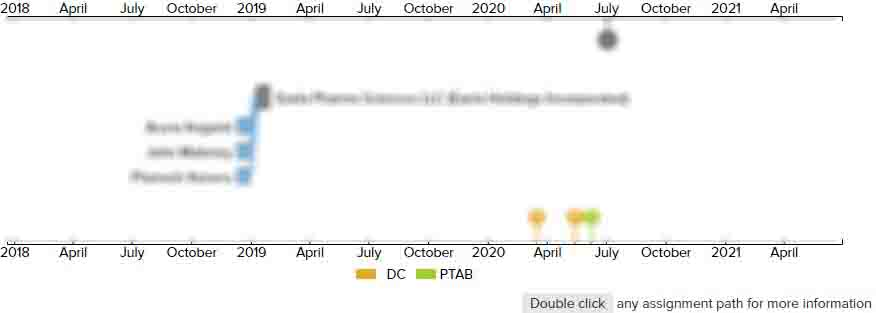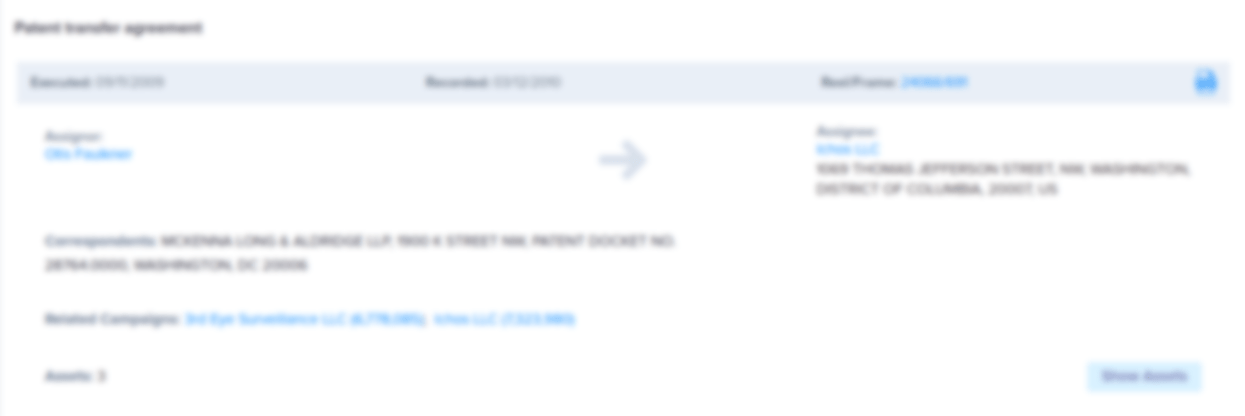# Magnetic absolute encoder

• US 7,471,080 B2
• Filed: 12/07/2007
• Issued: 12/30/2008
• Est. Priority Date: 12/28/2006
• Status: Active Grant
##### First Claim
Patent Images

1. A magnetic absolute encoder comprising:

• a magnetic sensor which outputs at least a pair of sine wave signals differing in phase by 90 degrees in association with the rotation of a multipolar-magnetized rotor;

main calculation means which detects the output of the magnetic sensor at a first detection period, and which calculates a single-rotation absolute value (1) that expresses the absolute position within one rotation of the rotor, and a multiple-rotation value (1) that expresses the number of rotations of the rotor from a preset origin;

backup calculation means which detects the output of the magnetic sensor at a second detection period that is longer than the first detection period, and which calculates a single-rotation absolute value (2) that expresses the absolute position within one rotation of the rotor, and a multiple-rotation value (2) that expresses the number of rotations of the rotor from the origin;

power supply control means which controls to drive the main calculation means and the backup calculation means using power supplied from a main power supply, and which controls to drive only the backup calculation means using power supplied from a backup power supply in cases where the main power supply is interrupted; and

an abnormality diagnosis means which compares the single-rotation absolute value (1) and single-rotation absolute value (2) respectively calculated by the main calculation means and the backup calculation means actuated by power from the main power supply, and which judges that an abnormality has occurred in cases where a state in which the difference between these values is equal to or greater than a predetermined difference continues for a specified number of rotations.

• ##### 1 Assignment
Timeline View
Assignment View×
×